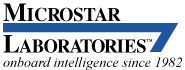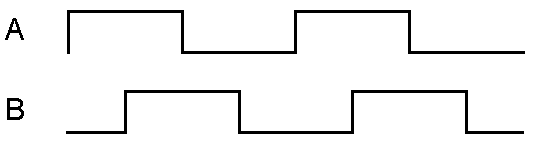Using the MSXB050 Quadrature Decoder Expansion Board

Technical Note TN-241 Version 1.00

Quadrature encoders are used to measure the angular position and speed of a rotating shaft. Figure 1 shows a quadrature encoded signal consisting of two squarewaves, called A and B, which are 90 degrees out of phase from each other. If A leads B by 90 degrees, the shaft is rotating clockwise, if A lags B by 90 degrees, the shaft is rotating counterclockwise. The rate of either squarewave depends on the rotational speed of the shaft.Figure 1. Typical quadrature encoded signal

The MSXB050 Quadrature Decoder expansion board can be used to extract the rotational speed, direction and relative position of up to four quadrature encoders. Connect the A and B outputs of the quadrature encoder to one of the "INxA" and "INxB". Connect the grounds to the "GNDx" terminals. If separate A and B grounds are not available, use a provided output ground or power ground.

The MSXB050 works by counting edge transitions on both the A or B input. Since the two signals are always 90 degrees out of phase, there are four edge transitions per cycle. For a quadrature encoded shaft having 10 cycles per degree (3600 pulses per revolution - a typical value), a full revolution provides a count of 360 * 10 * 4 = 14400 edge transitions. Clockwise rotation (where A leads B) results in an increasing count whereas counterclockwise rotation (A lags B) results in a decreasing count.

Since the MSXB050 uses 16-bit counters, in this example, the count will wrap around after slightly more than two revolutions. To get accurate, meaningful measurements, it is important to set the DAPL TIME value (the sampling period) small enough to prevent this. The actual value will depend on the expected maximum speed of the quadrature device. The MSXB050's counters are reset to zero at startup. A hardware jumper is available to reset all counters at any time, and a header is available that allows the individual counters to be reset by applying a signal which can be selected to be either a high or low logic level (refer to the MSXB050 manual). A quadrature encode often has another signal, called Z, which sends a pulse once per revolution. This is typically used for a zero degree reference and can also be used as the signal to reset the counter.

The DAPL commands QDCOUNT and CTRATE are used to determine the rotational data. QDCOUNT extends the 16-bit counter value obtained from the MSXB050 to a 32-bit value. This can be used to determine the accumulated number of revolutions that have occurred. QDCOUNT is a custom command that must be downloaded to the DAP before it can be used. It is currently found on the DAPtools installation CD in the "Extra\Msxb050\" directory. Select the version appropriate for the version of DAPL which is being used - typically DAPL2000 for all PCI and newer ISA boards, and DAPL4.x for older ISA boards. The file QDCOUNT.BIN must be downloaded to the DAP using DAPview for Windows, through the provided utility program comload.exe (for DAPL4.x) or cdload.exe (for DAPL2000), or through a separate user application.

CTRATE is a native DAPL command that returns the difference between the two successive samples. This tells directly the number of A and B edge transitions. This can be used to determine the number of revolutions and change in position since the last sample was taken, as well as the rotational speed of the shaft. The sign tells the direction of rotation: positive = clockwise, negative = counterclockwise.

The following DAPL example shows how to use QDCOUNT and CTRATE using the DAP's analog outputs to simulate a quadrature encoded signal. To test the operation of the MSXB050, open DAPview for Windows and copy this code into the DAP File window.

;MSXB050 Quadrature Decoder Board example program.
;
;Simulate a quadrature encoder signal using the two
; analog outputs available on the DAP.
;
;Setup:
; Attach an MSTB009 or MSXB037 to the DAP's analog I/O
; connector.  Attach an MSXB050 Quadrature Decoder
; Expansion board to the DAP's digital I/O connector.
;
; Connect the DAC0 and DAC1 outputs on the analog board
; to the IN0A and IN0B of the MSXB050 board.
;

RESET

;Declare two pipes to simulate a quadrature encoded signal.
PIPE PA, PB

;The outputs are staggered by the output clocking
; giving the following waveform.
;
;
;OClk:   � � � � � � � � � � � � � � � � � � � �
;AnOut0: 0   1   2   3   4   5   6   7   8   9
;AnOut1:   0   1   2   3   4   5   6   7   8   9
;
;        ____________________
;                   ___________________
;Lag:    __________|                   |________

; Use only one of the two sets of FILL commands.

; A leads B (CW rotation)
FILL PA 32767 32767 32767 32767 32767 0 0 0 0 0
FILL PB 0 0 32767 32767 32767 32767 32767 0 0 0

; A lags B (CCW rotation)
;FILL PB 32767 32767 32767 32767 32767 0 0 0 0 0
;FILL PA 0 0 32767 32767 32767 32767 32767 0 0 0

;Declare two pipes used for interpreting the returned data
PIPE PCNT LONG
PIPE PRATE

; Read the data from the MSXB050
IDEF IN1 2
SET IP0 B3   ;freeze the counter ports
SET IP1 B0   ;read the count from IN0A and IN0B
TIME 5000    ;each count read every (2 x 5000uSec)
END

PDEF A
;Generate A and B signals and send to analog outputs
REPLICATE (PA, 1, PA)
REPLICATE (PB, 1, PB)
COPY (PA, OP0)
COPY (PB, OP1)

;QDCOUNT maintains the count past the 16-bit transitions.
; The input procedure's TIME value must be low enough so
; that fewer than 32767 A/B edges occur per sample.
QDCOUNT(IP1, PCNT)

;CTRATE gives amount of change (in counts) per input sample.
; For the quadrature decoder board, one count is generated
; at every A and B edge transition.  This corresponds to
; the width of the 90 deg between the A and B inputs.
; Example: CTRATE gives 80, sampled at 10mSec. Edges are
; detected at a rate of: 10mSec/80=125uSec. Since A and B
; are the same frequency and 90 degrees apart, there are
; always 4 edges per cycle.  The means the rate of the
; A and B signals are 125 x 4 = 500uSec.  A 10 pulse per
; degree encoder would be rotating 500uSec x 3600 =
; 1.8 sec per revolution.
CTRATE(IP1, PRATE)

;Display the results
FORMAT COUNT=20 (IP1, PCNT, PRATE)
END

;Send the simulated quadrature waveforms to the analog
; outputs.  Note that the first "PB" output is delayed by
; one TIME value.
ODEF OUT2 2
SET OP0 A0   ;PA output
SET OP1 A1   ;PB output
TIME 25      ;period = 2 x 25uSec x 10 points = 500uSec
END

START
PAUSE 2000
STOP

;Notes:
;  Counter increments when A leads B,
;  decrements when B leads A

;  A positive CTRATE result means CW rotation,
;  negative means CCW.

View other Technical Notes.